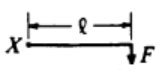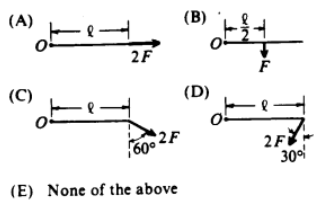# Problem: In which of the following diagrams is the torque about point O equal in magnitude to the torque about point X in the diagram above? (All forces lie in the plane of the paper.)

###### FREE Expert Solution

Torque equation:

$\overline{){\mathbit{\tau }}{\mathbf{=}}{\mathbit{r}}{\mathbit{F}}{\mathbf{sin}}{\mathbit{\theta }}}$ where θ is the nagle between r and F.

The torque about point X is:

τ = rFsinθ = (l)(F)sin90 = lF

97% (213 ratings)###### Problem DetailsIn which of the following diagrams is the torque about point O equal in magnitude to the torque about point X in the diagram above? (All forces lie in the plane of the paper.)